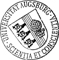## Convergence Analysis of an Adaptive Interior Penalty Discontinuous Galerkin Method for the Helmholtz Equation

• We are concerned with a convergence analysis of an adaptive Interior Penalty Discontinuous Galerkin (IPDG) method for the numerical solution of acoustic wave propagation problems as described by the Helmholtz equation. The mesh adaptivity relies on a residual-type a posteriori error estimator that does not only control the approximation error but also the consistency error caused by the nonconformity of the approach. As in the case of IPDG for standard second order elliptic boundary value problems, the convergence analysis is based on the reliability of the estimator, an estimator reduction property, and a quasi-orthogonality result. However, in contrast to the standard case, special attention has to be paid to a proper treatment of the lower order term in the equation containing the wavenumber which is taken care of by an Aubin-Nitsche type argument for the associated conforming finite element approximation. Numerical results are given for an interior Dirichlet problem and a screenWe are concerned with a convergence analysis of an adaptive Interior Penalty Discontinuous Galerkin (IPDG) method for the numerical solution of acoustic wave propagation problems as described by the Helmholtz equation. The mesh adaptivity relies on a residual-type a posteriori error estimator that does not only control the approximation error but also the consistency error caused by the nonconformity of the approach. As in the case of IPDG for standard second order elliptic boundary value problems, the convergence analysis is based on the reliability of the estimator, an estimator reduction property, and a quasi-orthogonality result. However, in contrast to the standard case, special attention has to be paid to a proper treatment of the lower order term in the equation containing the wavenumber which is taken care of by an Aubin-Nitsche type argument for the associated conforming finite element approximation. Numerical results are given for an interior Dirichlet problem and a screen problem illustrating the performance of the adaptive IPDG method.• Dokument_1.pdfAuthor: Ronald H. W. HoppeGND, Natasha S. Sharma urn:nbn:de:bvb:384-opus4-12577 https://opus.bibliothek.uni-augsburg.de/opus4/1581 Preprints des Instituts für Mathematik der Universität Augsburg (2011-15) Preprint English Universität Augsburg University of Houston 2011/10/17 Discontinuous Galerkin; Interior Penalty; Helmholtz equation; adaptivity; convergence analysis Finite-Elemente-Methode; Diskontinuierliche Galerkin-Methode; Helmholtz-Schwingungsgleichung; Konvergenz Mathematisch-Naturwissenschaftlich-Technische Fakultät Mathematisch-Naturwissenschaftlich-Technische Fakultät / Institut für Mathematik Mathematisch-Naturwissenschaftlich-Technische Fakultät / Institut für Mathematik / Lehrstuhl für Numerische Mathematik 5 Naturwissenschaften und Mathematik / 51 Mathematik / 510 MathematikDeutsches Urheberrecht mit Print on Demand